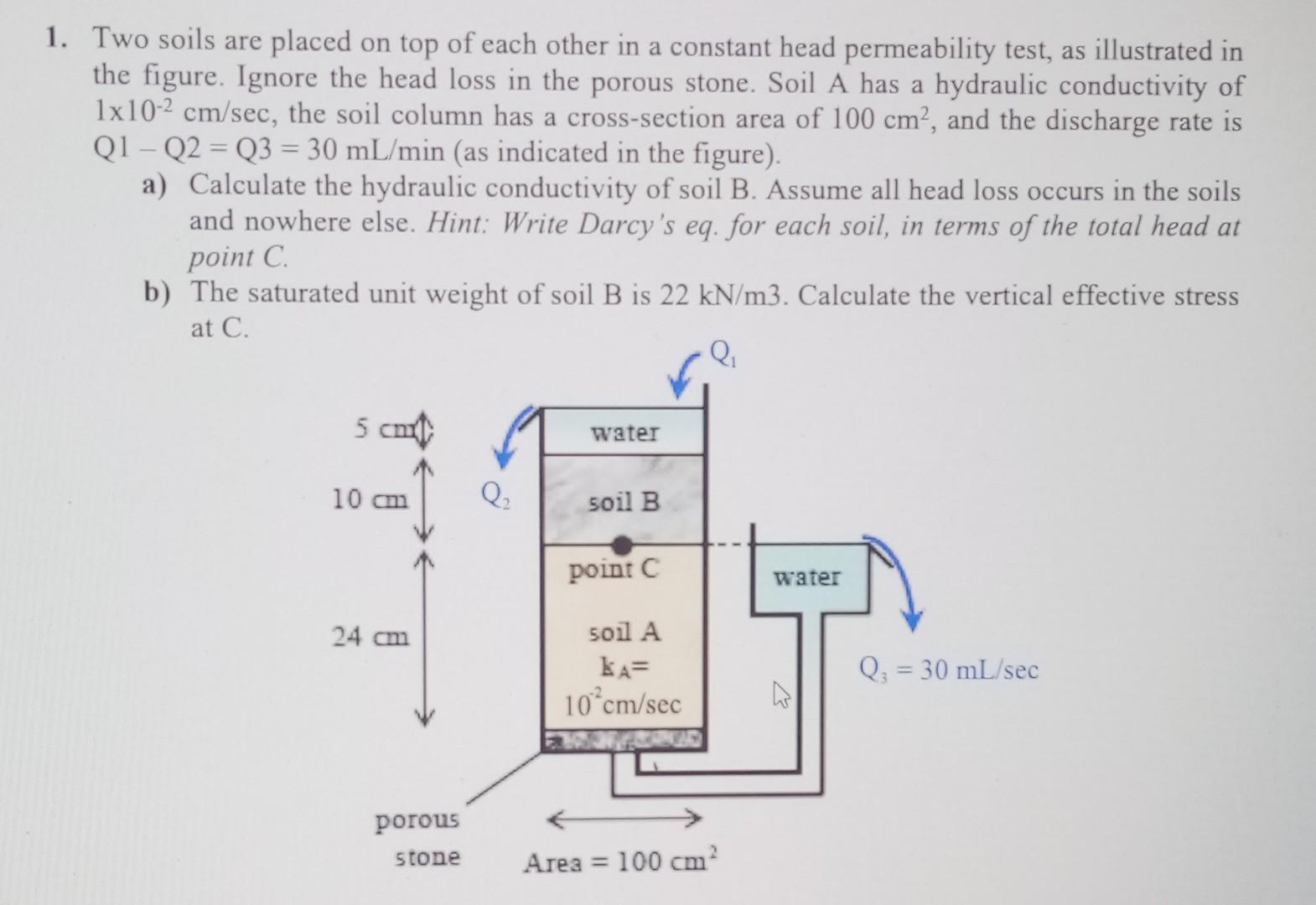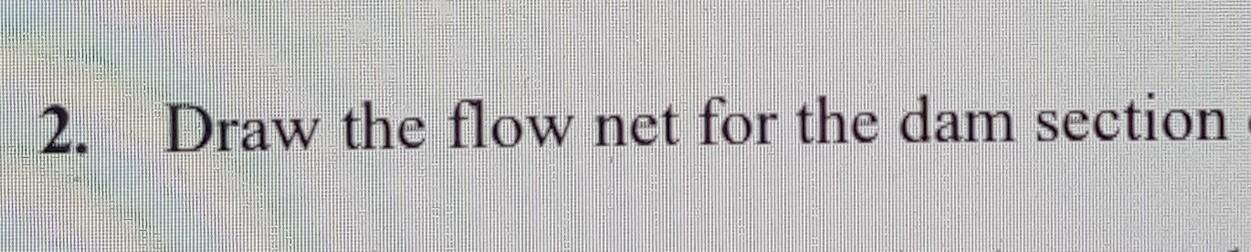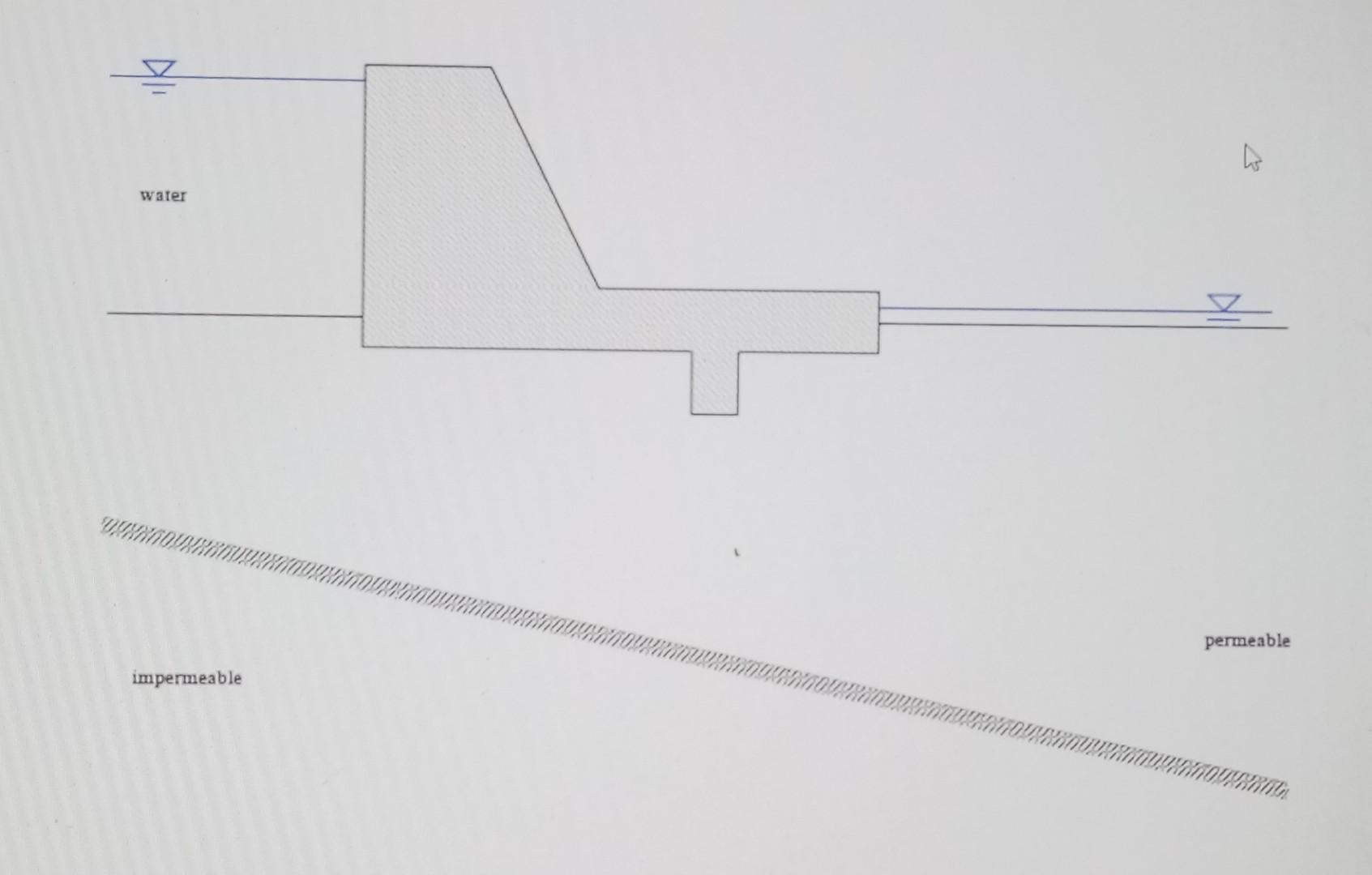# (Solved): These questions are the questions of the soil mechanics course. Please do these two questions. ...

These questions are the questions of the soil mechanics course. Please do these two questions.Two soils are placed on top of each other in a constant head permeability test, as illustrated in the figure. Ignore the head loss in the porous stone. Soil A has a hydraulic conductivity of , the soil column has a cross-section area of , and the discharge rate is Q1 - Q2 = Q3 = (as indicated in the figure). a) Calculate the hydraulic conductivity of soil B. Assume all head loss occurs in the soils and nowhere else. Hint: Write Darcy's eq. for each soil, in terms of the total head at point . b) The saturated unit weight of soil is . Calculate the vertical effective stress at C. 2. Draw the flow net for the dam section permeable impermeable

We have an Answer from Expert